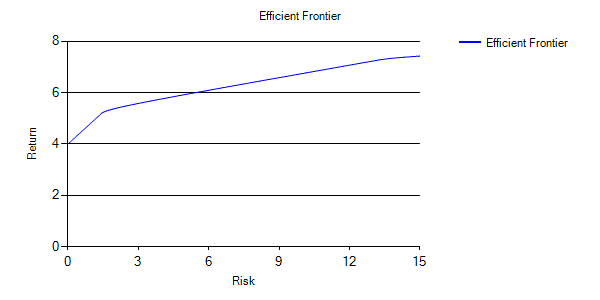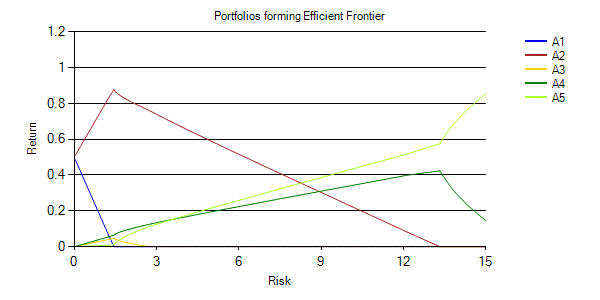/

### Extreme Events – Specimen Question A.5.1(b) – Answer/Hints

Q. Show how the efficient frontier and the asset mixes making up the points along the efficient frontier would alter if risk-free is equated with 50% in Asset A1 and 50% in Asset A2.

Any of the approaches used to answer Question A.5.1(a) can be used to answer this question. If you are using the Nematrian online toolkit (perhaps in conjunction with its SmartChart facility) then the only thing you need to change is to alter the Minimum Risk Portfolio so that it is now {0.5, 0.5, 0, 0, 0}, in which case the answer is:

Efficient frontier:Portfolios forming the efficient frontier